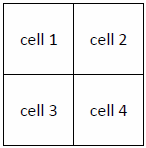# [ASK] which is the best way for doing a looping

as we know ionic used a grid system which the code is like:

``````<div class="row">
<div class="col">
...
</div>
<div class="col">
...
</div>
</div>
``````

so how i do a looping if the output would be like this, if i get the data from ng-repeat. Is that possible if i use `(2%2==0) or modulus` with angular script?